Introducing the statistics of risk

Translate the power of knowledge into action. Open Free* Demat Account

# The variance and covariance matrix

4.2In an earlier chapter, we computed the covariance for a two-stock portfolio. Now, that is simple, because there are only 2 stocks. But say we have a five-stock portfolio, comprising stocks A, B, C, D and E. In that case, we have to calculate the covariance for the following pairs:

1. A and B
2. A and C
3. A and D
4. A and E
5. B and C
6. B and D
7. B and E
8. C and D
9. C and E
10. D and E

So, for a five-stock portfolio, we need to calculate 12 covariances before proceeding to calculate the variance and risk. This gets more complex as the size of the portfolio increases. And you know that a good portfolio is one that is well-diversified. So, if you have a portfolio of 10 to 12 assets, calculating the covariance there is definitely going to be cumbersome. Here’s where matrices and good old excel can be of help.

These tools can help us construct a variance-covariance matrix (often just written as variance covariance matrix). And that is the first step to calculating the risk for a portfolio.

### What is a variance-covariance matrix?

In the context of investment-related risk calculations, a variance-covariance matrix is a rectangular matrix that contains the variances and covariances of the stocks in a portfolio. The diagonal elements of the matrix represent the variances of the individual stocks. The other elements represent the covariances between the different pairs of stocks in the portfolio. This will become clearer after we see how to create the matrix.

### Steps involved in creating the variance-covariance matrix

There is a simple formula to create this matrix for a portfolio with z number of stocks, with n number of observations:

 Matrix z x z = (1 ÷ n) XT X

Here’s what the formula means:

• z is the number of stocks in the portfolio
• Matrix z x z is the variance-covariance matrix
• n is the number of observations taken
• X is the excess return matrix
• XT is the transpose of matrix X

On its own, these things can be confusing. That’s why we’ll take up an example, as usual, to understand it better.

First, let’s look at the step-by-step guide to create this matrix.

1. Calculate the daily returns from each stock in your portfolio.
2. Calculate the average daily returns from each stock.
3. Compute the difference between the daily return and the average return - for each stock, of course. This is the excess return, and it will give you the excess return matrix we saw in the formula above.
4. Set up XT, which is the transpose of the excess return matrix.
5. Multiple matrix X with matrix XT.
6. Divide each element in the XT X matrix by n.

So, that sums up the theory of the steps involved. Let’s take a look at an example.  We’ll take a small portfolio - made of just 3 stocks - to begin with. And as we did in the chapter before the last one, we’ll take just 10 days’ worth of observations to keep the calculations easy to understand.

### Creating the variance-covariance matrix: An example

We’ll take a set of hypothetical prices for the following stocks over a 10-day period: stock A, stock B, and stock C. The first two, we’d already seen them in the third chapter. So, we’ll just add a third stock to the set to create a 3-stock portfolio. Here are the prices for these stocks over 10 days.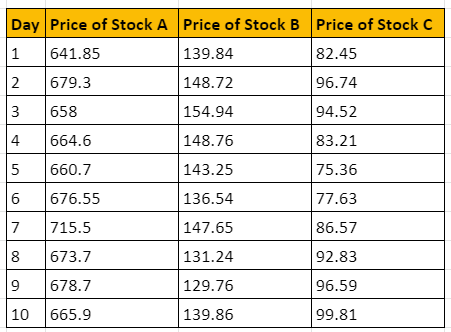Now, we’ll begin the step-by-step process we saw earlier.

### Step 1: Calculate the daily returns from each stock in your portfolio

This is simply the difference in return between two days, divided by the base return. Check out the daily returns for the three stocks. From here on, we’ll only need to concern ourselves with this. The price of the stock does not feature in our process any more.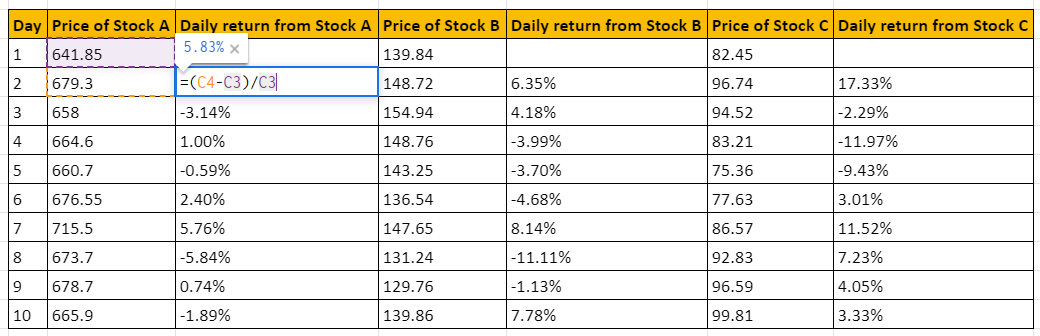### Step 2: Calculate the average daily returns from each stock

This is easy. You just need to use the average function in the excel software. Here’s how it goes for our stocks.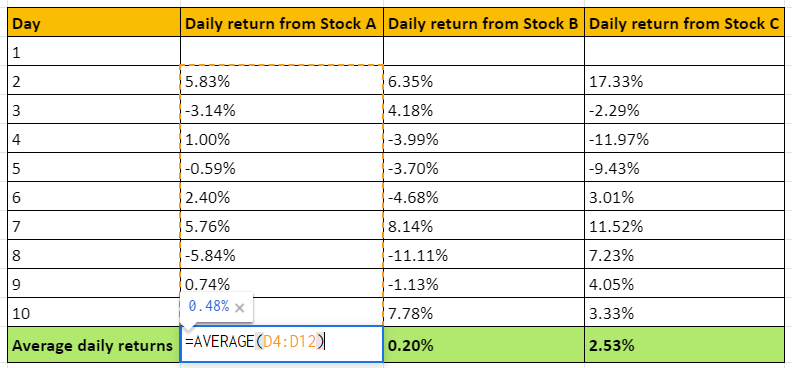So, the average returns for the three stocks come in as:

• Stock A: 0.48%
• Stock B: 0.20%
• Stock C: 2.53%

### Step 3: Compute the excess return and set up the excess return matrix X

The excess return is basically the difference between each day’s return and the average return of each stock. In other words, it shows by how much each day’s return exceeds (or falls short of) the average returns.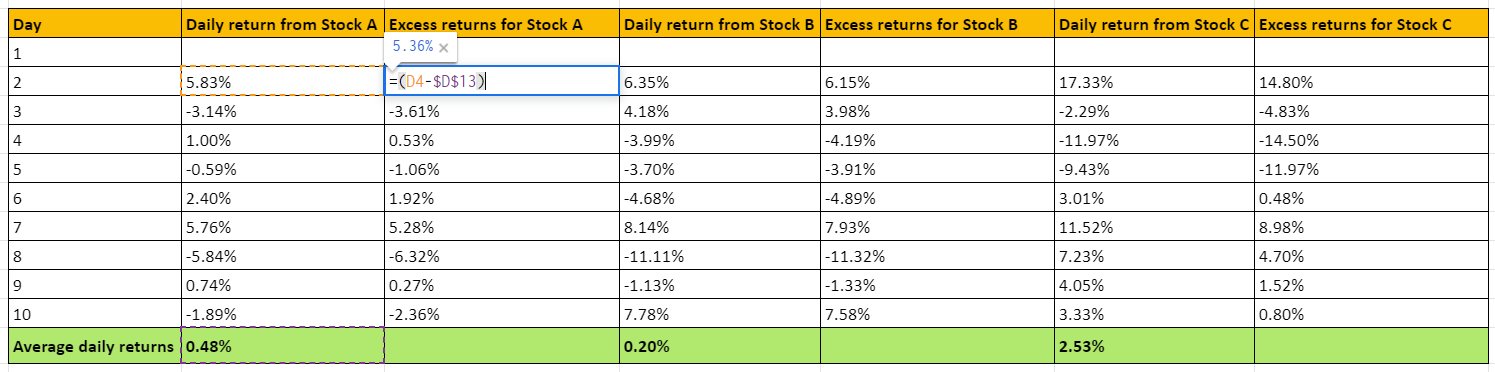To set up the excess return matrix, we just need to take the values of the excess returns and place them together to form the array. Check it out below.

Matrix X: The excess return matrix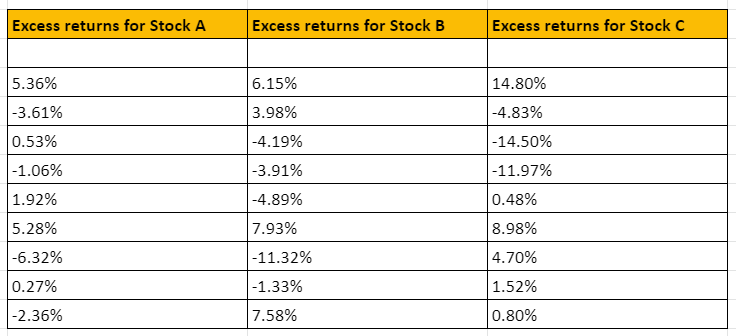The size of this matrix is n x z, where n is the number of observations and z is the number of stocks. So, this is a 9 x 3 matrix.

### Step 4: Set up XT, which is the transpose of the excess return matrix

Remember we discussed transposes of matrices in the previous chapter? It’s essentially just flipping the rows and columns of the original matrix. So, the transpose of matrix X is matrix XT, which looks like this.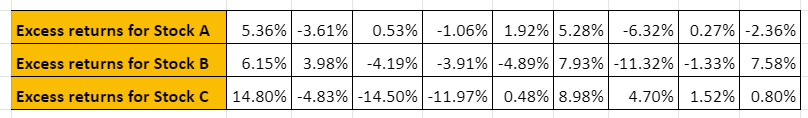The size of this matrix is z x n, where z is the number of stocks and n is the number of observations. So, this is a 3 x 9 matrix.

### Step 5: Multiple matrix X with matrix XT

Recall matrix multiplication from the previous chapter. The fundamental rule to make matrix multiplication possible is that the number of columns in the first matrix must match the number of rows in the second matrix. And so far, these are two matrices we have.

• Matrix X, which is a 9 x 3 matrix
• Matrix XT, which is a 3 x 9 matrix

Technically, we can multiply each with the other. But recall the formula we saw at the beginning of the chapter? The variance-covariance matrix is formed as follows:

 Matrix z x z = (1 ÷ n) XT X

So, we need to multiply X transpose with X. Again, there’s a function in excel for this. It’s called MMULT. And using it, this is what we get as the product.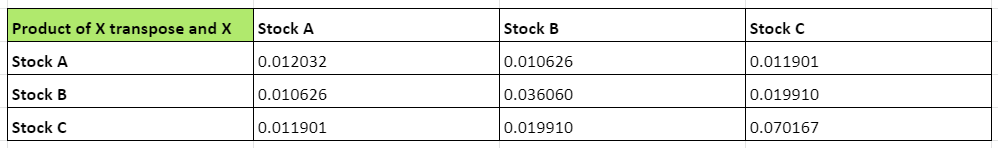This matrix is formed by multiplying a 3 x 9 matrix and a 9 x 3 matrix. So, its size will be 3 x 3. Also, this is not the variance-covariance matrix by itself. There is still one more step to go through.

### Step 6: Divide each element in the XT X matrix by n

Here, n is 9, which is the number of observations we’ve taken. Let’s divide the matrix by 9 to get the variance-covariance matrix. This basically means we divide each element by 9. Check out the result here.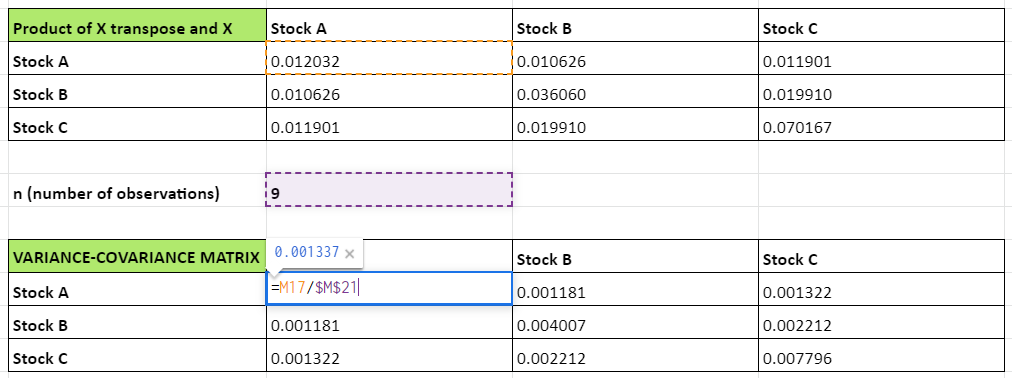### Understanding the variance-covariance matrix

Alright, so, the calculations are done. But what does the variance-covariance matrix indicate? And why is it named this way, in the first place? Let’s try and decode it before we move on.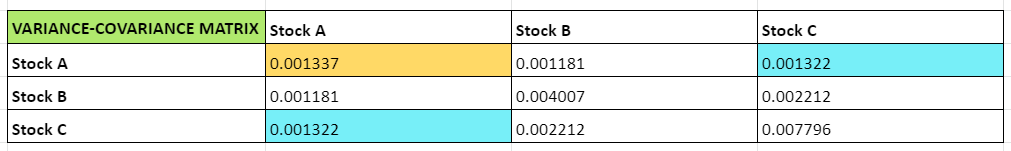See how the matrix has turned out? The numbers are very small, in decimals, of course. But what do they indicate? Simply put, they tell you the covariance between two stocks. For example, take stocks A and C.

The covariance of stock A with stock C will be the same as the covariance of stock C with stock A. In the image above, this value has been highlighted in the two blue cells: 0.001322. Similarly, take the cell coloured yellow. What does that indicate? The covariance of stock A with itself? That is basically the variance.

Here, it is 0.001337 for stock A, 0.004007 for stock A and 0.007796for stock C.

If you directly use the variance formula in excel, you’ll get the same values. See below.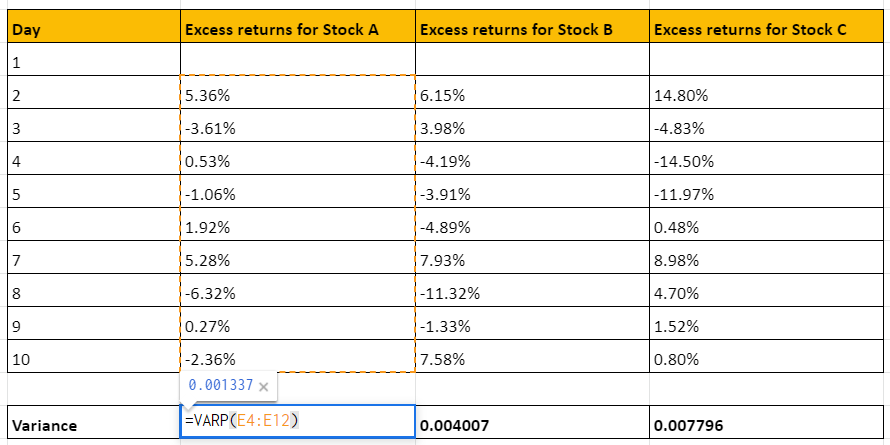So, since this matrix gives you the variance of each asset, and the covariance of each possible pair of assets, it’s called the variance-covariance matrix.

#### Wrapping up

Now, what is the purpose of this matrix? Does it give us the portfolio variance? Not really, no. Instead, it is a stepping stone to the correlation matrix, which we’ll be discussing in the next chapter. Keep reading to complete the last leg of this journey.

#### A quick recap

• Calculating the covariance between assets gets more complex as the size of the portfolio increases.
• But matrices and good old excel can be of help.
• There is a simple formula to create this matrix for a portfolio with z number of stocks, with n number of observations: Matrix z x z = (1 ÷ n) XT X
• Once you set up this matrix, you can get the variance of each stock and the covariance of each pair of stocks at a glance.